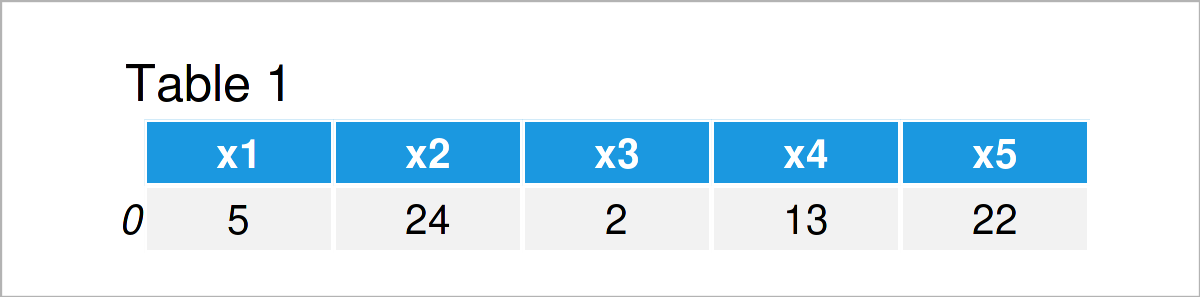# Convert Dictionary to pandas DataFrame in Python (Example)

In this Python article you’ll learn how to create a pandas DataFrame from a dictionary.

The article will consist of one example for the creation of a pandas DataFrame from a dictionary. More precisely, the tutorial looks as follows:

Let’s dig in!

## Creation of Example Data

The following dictionary is used as a basis for this Python programming tutorial.

```my_dict = {'x1': 5,                  # Create example dictionary
'x2': 24,
'x3': 2,
'x4': 13,
'x5': 22}
print(my_dict)                       # Print example dictionary
# {'x1': 5, 'x2': 24, 'x3': 2, 'x4': 13, 'x5': 22}```

As you can see, our example dict contains five key and value pairs. Let’s convert this dictionary to a pandas DataFrame!

## Example: Create pandas DataFrame from Dictionary

This example shows how to construct a pandas DataFrame based on a dictionary in the Python programming language.

In order to use the functions and commands of the pandas library, we first have to load pandas:

`import pandas as pd                  # Load pandas library`

Next, we can apply the DataFrame function of the pandas library to our dictionary to transform it into a DataFrame object. Note that square brackets around the name of the dictionary:

```my_data = pd.DataFrame([my_dict])    # Apply DataFrame() function
print(my_data)                       # Print pandas DataFrame```Table 1 shows that the pandas DataFrame that we have created from our dict consists of one row and five columns.

## Video, Further Resources & Summary

Do you need more information on the Python programming syntax of this tutorial? Then you might want to watch the following video on my YouTube channel. I explain the Python code of this tutorial in the video:

Please accept YouTube cookies to play this video. By accepting you will be accessing content from YouTube, a service provided by an external third party.If you accept this notice, your choice will be saved and the page will refresh.

Besides that, you could have a look at some of the related Python articles on www.statisticsglobe.com:

At this point of the post you should have learned how to convert a dictionary to a pandas DataFrame in the Python programming language. Please let me know in the comments section, if you have additional questions or comments. Furthermore, please subscribe to my email newsletter in order to get updates on the newest articles.

Subscribe to the Statistics Globe Newsletter